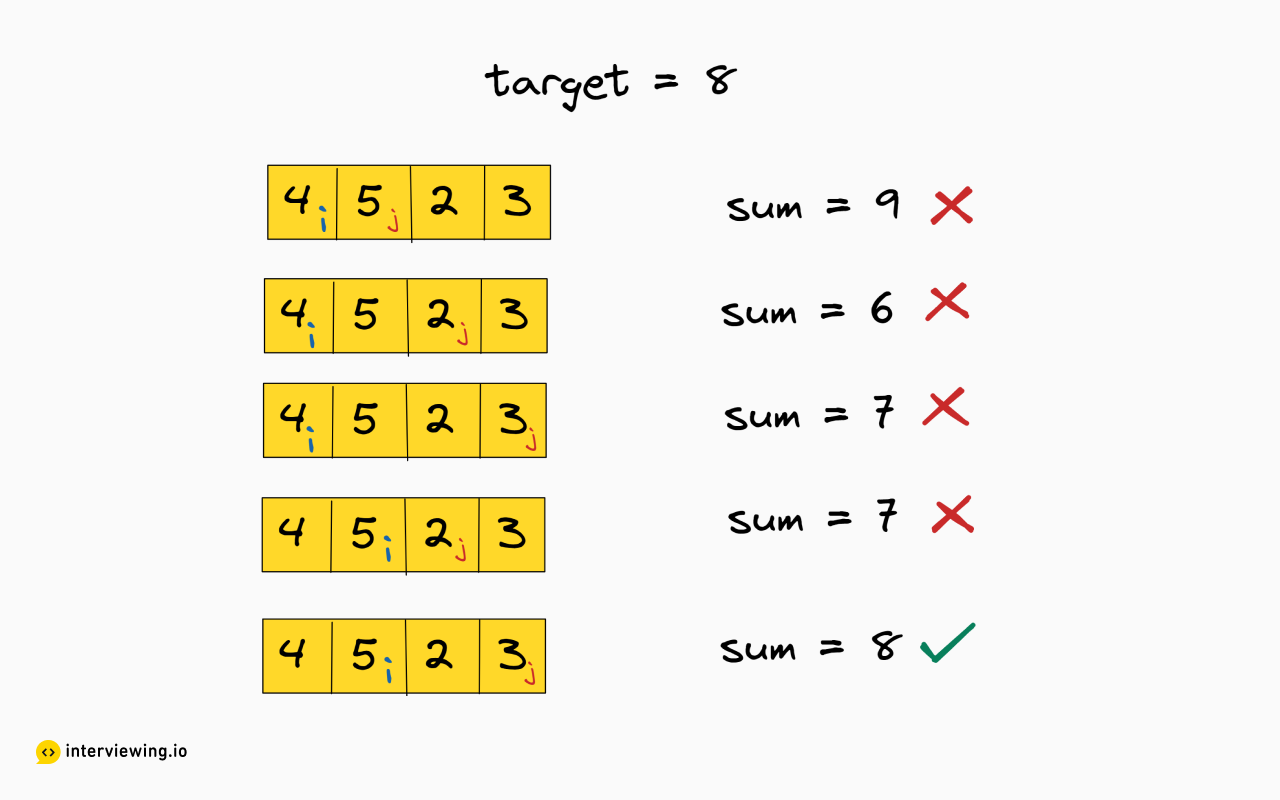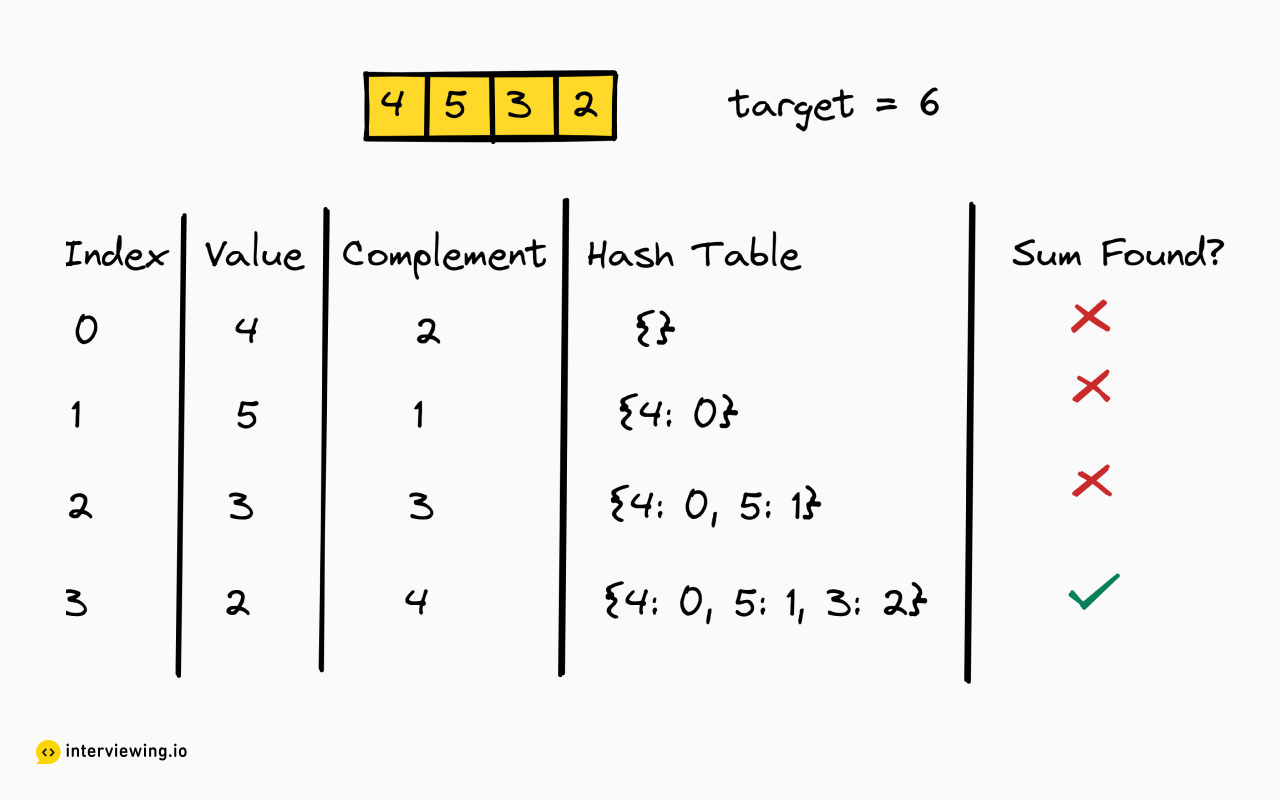EASY
DATA STRUCTURES AND ALGORITHMS

# How to Solve Two Sum

Written ByTom WagnerDominic Platt

## Two Sum Introduction

The Two Sum problem involves finding two numbers in an array that add up to a given target number. This is a classic problem whose solution progresses naturally from a less efficient, iterative approach, to a more efficient algorithm enabled through the use of sorting and hashing data structures. Before viewing the problem and solution, below are some short video snippets from real mock interviews to help prepare you for some common pitfalls that interviewees stumble into.

### • Interview Analysis: Snippets from Real Interviews 🔥

1. Common Coding Mistakes: Returning Result
The importance of remembering to add a return statement

2. Common Coding Mistakes: Calculation Error
Candidates always make this simple mistake when calculating the difference between the target value and input numbers

3. Common Coding Mistakes: Calculation Error
Look out for this when dealing with boolean values.

4. Common Python Mistakes: Case Sensitive Syntax Error
Python is case-sensitive, you'll want to avoid the mistake this candidate made.

5. Analysis: Edge cases
Covering edge cases before coding is standard procedure, don't find yourself in an awkward position by missing this simple edge case in your next interview.

6. Analysis: Time and Space Complexity
A senior engineer expertly explains how to reach the optimal time and space complexity for the two-sum problem.

## Two Sum Problem

Given an array of integers, return the indices of the two numbers that add up to a given target.

### Example Inputs and Outputs

#### Example 1

Input: nums = [5, 2, 3], target = 8 Output: [0, 2]

#### Example 2

Input: nums = [3, 2, 4], target = 6 Output: [1, 2]

#### Example 3

Input: nums = [5, 5], target = 10 Output: [0, 1]

Constraints

• There is always exactly one solution

## Two Sum Solutions

#### Approach 1: Brute Force

We need to find the combination of which two numbers add up to a given target. We can split this into two steps: 1.) Iterate over every possible pair of numbers 2.) Check if a given pair sums up to our target.

How do we iterate over every pair of numbers? We can start with the first number and compare it against every other number in the array. Then we move the next number and compare it against every other number and so on until we have found our solution.

We can then easily check if a given pair adds up to our target. If it does then we have found our solution and we can return the two indices.From the diagram, we can see this approach in action. Where `i` and `j` represent the index pairs. On each iteration, we calculate the sum and see if it is equal to our target. Notice how `j` is never behind `i`, which ensures that we never re-evaluate already evaluated sums.

#### Two Sum Python, JavaScript and Java Solution - Brute Force

``````class Solution:
def twoSum(self, nums: List[int], target: int) -> List[int]:
# 1. Iterate over every possible number pair
for i in range(len(nums)):
# j is always ahead of i so that we don't re-evaluate already evaluated sums
for j in range(i+1, len(nums)):
# 2. Check if a given pair adds up to our target
if nums[i] + nums[j] == target:
# Return the indices when a pair has been found
return i, j``````

#### Time / Space Complexity Analysis

• Time Complexity: `O(n<sup>2</sup>)`, where `n` is the size of the array. For each number, we have to evaluate every other number in the array.

• Space Complexity: `O(1)`, disregarding the input, all other variables have constant space.

#### Approach 2: Hash Table

We can build on the brute force solution and see that there is a lot of repeated work. This is because we iterate over the array multiple times.

Why do we need to iterate multiple times? For each number, we try to find another number which sums to the target. We refer to this other number as the "complement", and to find this number we check every other element in the array.

We can rephrase the previous algorithm: 1.) Iterate over every number in the array 2.) For each number scan the rest of the array to see if there is another number which sums to the target.

Notice how we repeatedly search the array for this "complement" number (step #2 above), which takes `O(n)` time. Is there some way we can do this step faster?

We can reduce this repeated work by using a hash table. A hash table is a data structure which stores key-value pairs, a value can be looked up with a given key in constant time. We can store a number with its index in the hash table. Then when we need to check if the complement number is in the array, we can do so in constant time.

With this in mind we can break down our hash table algorithm into steps:

1.) Iterate over every number in the array This can be done with a simple for loop.

2.) Calculate the complement The complement is the other number that would sum to the target and can be calculated as `target - num`.

3.) Check if that complement is in our hash table If the complement is in the hash table then we can look up its index and we have our solution! If it is not then we continue to search the array.

4.) Add the current number to our hash table The current number may sum up with another number that we have not yet evaluated. We can store the number in the hash table as a key with the index as the value for future checks.From the diagram, we can see how the hash table is modified and evaluated through each iteration until we find a sum.

#### Two Sum Python, JavaScript and Java Solution - Hash Table

``````class Solution:
def twoSum(self, nums: List[int], target: int) -> List[int]:
# Our hash table that stores at which index the number is at
numToIndex = {}

# 1. Iterate over every number in the array
for i in range(len(nums)):
# 2. Calculate the complement that would sum to our target
complement = target - nums[i]

# 3. Check if that complement is in our hash table
if complement in numToIndex:
return numToIndex[complement], i

# 4. Add the current number to our hash table
numToIndex[nums[i]] = i``````

#### Time/Space Complexity

• Time Complexity: `O(n)`, where `n` is the size of the array. We iterate over every number in the array and the hash table lookup/add operations take constant time.
• Space Complexity: `O(n)`, where `n` is the size of the array. Our hash map stores every number in the input array.

## Practice the Two Sum Problem With Our AI Interviewer

Start AI Interview

## Two Sum Analysis

#### Analysis: Time and Space Complexity

In this snippet, the candidate explains the time and space complexity for his proposed solution using merge sort. The interviewer expertly highlights how sorting doesn't buy you much when tackling the two sum problem and instead explains how a map would provide you with the O(n) time in an optimized solution. Another key element to note is that two sum problem requires you to print out the pairs whereas this candidate returns a list incorrectly.

#### Common Coding Mistakes: Returning Result

A common mistake to look out for is forgetting to return or pass values out of your functions. In this snippet the candidate ran the code which returned "none". This was fixed by adding a return statement for results.

#### Common Python Mistakes: Case Sensitive Syntax Error

A common mistake candidates might make when working in Python is forgetting to capitalize the "T" for True as Python is case sensitive. In Python, built-in constants such as True, False, None, NotImplemented and Ellipsis all require a capital letter at the beginning.

#### Analysis: Edge cases

In this snippet, the candidate discovers an edge case that causes his solution to fail after already having coded up the full solution. As a result the interviewer highlights the importance of identifying and asking about edge cases such as duplicates and checking if both inputs are valid. These edge cases should be covered before beginning to code.

#### Common Coding Mistakes: Calculation Error

A common mistake during the two sum problem is incorrectly assuming numbers that subtract together to reach the target value are valid pairs. In this case with a target of 3, the numbers 13 and 10 would not count as a valid pair, this mistake can occur when calculating target compliment. The correct order would be to have target - value as you want to find the two numbers that add up to the target number.

#### Common Coding Mistakes: Calculation Error

An important point to remember when doing calculations with variables is that if these variables have been defined as a Boolean either True of False, they will have a value attached to them. In this case "value_dict[value]" was defined as True. When calculating target compliment, "value_dict[value]" was being treated as equalling 0. This resulted in the output incorrectly returning -2 over and over. Be on the look out for this common mistake when working with Boolean values.